It’s World Statistics Day! To honor the theme of the day, the JMP User Community is having conversations about the importance of trust in statistics and data. And we want to hear from you! Tell us the steps you take to ensure that your data is trustworthy.
Choose Language Hide Translation Bar
Highlighted

## Connecting data points using JSL

Hi

I am trying to connect data points within a graph in certain order (like creating diamond plot etc.). When I connect manually using line and export the script, I see that it used line function with (X,Y) coordinate. Can anyone please help me with

1. I assume those (X,Y) are frame coordinate?

2. Given 1. is true, How do I find out those (X,Y) using JSL? More specifically, need to know the syntax on how to extract out frame (X,Y) for each data point in the graph?

Any other efficient way you know how to connect data points in JMP?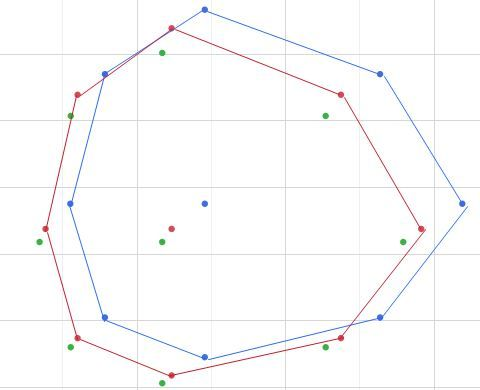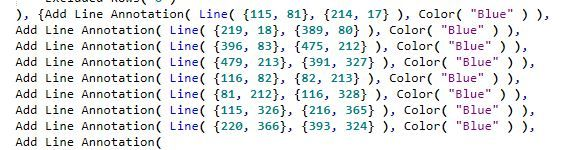Thanks much

2 ACCEPTED SOLUTIONS

Accepted Solutions
Highlighted

## Re: Connecting data points using JSL

I have adjusted your script a bit, but the main issue is that your code is assuming that you have all combinations of Group1, Group2 and Group3, and in the data you supplied you do not, so the program can not find any data to analyze.

``````names default to here(1);

dtt = Current Data Table();

Summarize( dtt, byGroup1 = by( :var1 ) );
Summarize( dtt, byGroup2 = by( :var2 ) );
Summarize( dtt, byGroup3 = by( :var3 ) );

For( ii = 1, ii < N Items( byGroup1 ), ii++,
For( jj = 1, jj < N Items( byGroup2 ), jj++,
For( kk = 1, kk < N Items( byGroup3 ), kk++,
dtt << select where( :var1 == byGroup1[ii] & :var2 == byGroup2[jj] & :var3 == byGroup3[kk] );
show(ii,jj,kk,bygroup1[ii],bygroup2[jj],bygroup3[kk]);
sel = dtt << get selected rows;
If( n rows(sel) > 0,
show("inside",ii,jj,kk);
dt = dtt << subset( selected rows(1), selected columns(0));
gb = dt << Graph Builder(
Size( 531, 456 ),
Show Control Panel( 0 ),
Variables( X( :x axis ), Y( :y axis ), Overlay( :legend ) ),
Elements( Points( X, Y, Legend( 5 ) ) ),
SendToReport(
Dispatch(
{},
"x axis",
ScaleBox,
{Format( "Best", 12 ), Min( 2.273568 ), Max( 3.00134902479339 ), Inc( 0.1 ), Minor Ticks( 0 )}
)
)
);
// Add a simple graphics script to connect the points
Report( gb )[FrameBox( 1 )] << add graphics script(
Summarize( dt, byGroup = by( :legend ) );
colorList = {"Blue", "Red", "Green"};
Fill Pattern(  );
For( i = 1, i <= N Items( byGroup ), i++,
Pen Color( colorList[i] );
theRows = dt << get rows where( :legend == byGroup[i] & :Label != "i" );
// Add the first value as the last value to complete the object
theRows = theRows |/ theRows;
xMatrix = :x axis[theRows];
yMatrix = :y axis[theRows];
Line( xMatrix, yMatrix );
);
););
)
)
);``````
Jim
Highlighted

## Re: Connecting data points using JSL

No word to appreciate all your help. Thanks a million.

8 REPLIES 8
Highlighted

## Re: Connecting data points using JSL

First, see Help > Scripting Index and search for the Line() function. Then go to Help > JMP Documentation Library > Scripting Guide and search for it again for a more detailed explanation and more examples.

Learn it once, use it forever!
Highlighted

## Re: Connecting data points using JSL

Thanks for your input but I was not able to find out what I was looking for. I see different line function and it shows all for a given coordinate. My question was more on how to find those coordinate [frame and not data (x,y)] for a given data point in the graph. I was able to connect using graph builder but then it also connect the center data points which I do not want. If I do exclude then those data points are not shown which also I do not want. I can still replicate the first set of data points to close the diamond but then do not know how I can still show the data points in the center but not connecting it. Thanks much.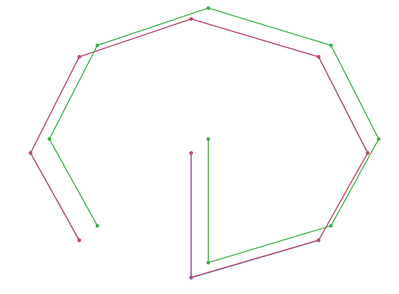Highlighted

## Re: Connecting data points using JSL

Can you please supply the data that you are creating the graphs from?

Jim
Highlighted

## Re: Connecting data points using JSL

here is the data

 x-axis y-axis label legend 2.3856 2.2608 a set1 2.3858 2.2616 a set2 2.4184 2.2919 a set3 2.2979 2.4459 b set1 2.2979 2.4463 b set2 2.3318 2.4758 b set3 2.3856 2.6498 c set1 2.3858 2.6499 c set2 2.4184 2.6742 c set3 2.5875 2.7302 d set1 2.5878 2.7302 d set2 2.6185 2.7532 d set3 2.8174 2.6498 e set1 2.8177 2.6499 e set2 2.8398 2.6742 e set3 2.9059 2.4459 f set1 2.9062 2.4463 f set2 2.9259 2.4758 f set3 2.8174 2.2608 g set1 2.8177 2.2616 g set2 2.8398 2.2919 g set3 2.5875 2.1817 h set1 2.5878 2.1827 h set2 2.6185 2.2138 h set3 2.5875 2.4459 i set1 2.5878 2.4463 i set2 2.6185 2.4758 i set3

I am trying to connect data points for each set but not including "i" group (the center data). sample snapshot is below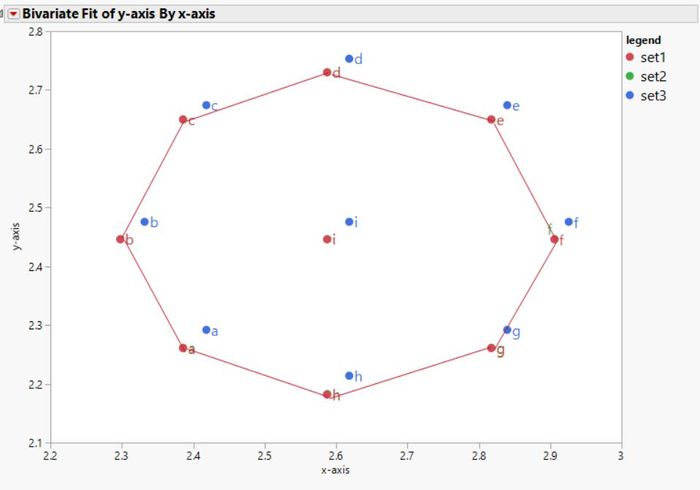Highlighted

## Re: Connecting data points using JSL

If a simple graphics script is added to the Graph Builder object you can get what you want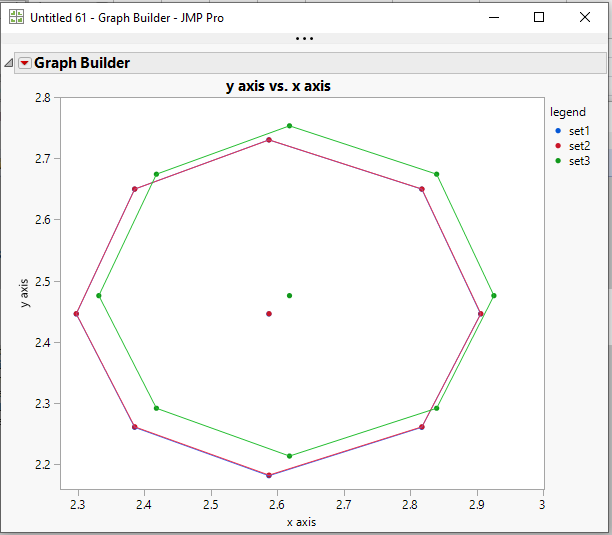``````names default to here(1);

// Create the data table
dt = New Table( "Example",
New Script(
"Source",
Data Table( "Untitled 60" ) << Split(
Split By( :Column 2 ),
Split( :Column 1 ),
Sort by Column Property
)
),
New Column( "x axis",
Numeric,
"Continuous",
Format( "Best", 12 ),
Set Values(
[2.3856, 2.3858, 2.4184, 2.2979, 2.2979, 2.3318, 2.3856, 2.3858, 2.4184,
2.5875, 2.5878, 2.6185, 2.8174, 2.8177, 2.8398, 2.9059, 2.9062, 2.9259,
2.8174, 2.8177, 2.8398, 2.5875, 2.5878, 2.6185, 2.5875, 2.5878, 2.6185]
)
),
New Column( "y axis",
Numeric,
"Continuous",
Format( "Best", 12 ),
Set Values(
[2.2608, 2.2616, 2.2919, 2.4459, 2.4463, 2.4758, 2.6498, 2.6499, 2.6742,
2.7302, 2.7302, 2.7532, 2.6498, 2.6499, 2.6742, 2.4459, 2.4463, 2.4758,
2.2608, 2.2616, 2.2919, 2.1817, 2.1827, 2.2138, 2.4459, 2.4463, 2.4758]
)
),
New Column( "label",
Character,
"Nominal",
Set Values(
{"a", "a", "a", "b", "b", "b", "c", "c", "c", "d", "d", "d", "e", "e",
"e", "f", "f", "f", "g", "g", "g", "h", "h", "h", "i", "i", "i"}
)
),
New Column( "legend",
Character,
"Nominal",
Set Values(
{"set1", "set2", "set3", "set1", "set2", "set3", "set1", "set2", "set3",
"set1", "set2", "set3", "set1", "set2", "set3", "set1", "set2", "set3",
"set1", "set2", "set3", "set1", "set2", "set3", "set1", "set2", "set3"}
)
)
);

// Draw the scatterplot
gb = dt << Graph Builder(
Size( 531, 456 ),
Show Control Panel( 0 ),
Variables( X( :x axis ), Y( :y axis ), Overlay( :legend ) ),
Elements( Points( X, Y, Legend( 5 ) ) ),
SendToReport(
Dispatch(
{},
"x axis",
ScaleBox,
{Format( "Best", 12 ), Min( 2.273568 ), Max( 3.00134902479339 ),
Inc( 0.1 ), Minor Ticks( 0 )}
)
)
);
// Add a simple graphics script to connect the points
Report( gb )[FrameBox( 1 )] << add graphics script(
Summarize( dt, byGroup = by( :legend ) );
colorList = {"Blue","Red","Green"};
fill pattern(  );
For( i = 1, i <= N Items( byGroup ), i++,
Pen Color(colorList[i]);
theRows = dt << get rows where( :legend == byGroup[i] & :Label != "i" );
// Add the first value as the last value to complete the object
theRows = theRows |/ theRows;
xMatrix = :x axis[theRows];
yMatrix = :y axis[theRows];
line( xMatrix, yMatrix );
);
);

``````
Jim
Highlighted

## Re: Connecting data points using JSL

Thank you so very much for the help. Greatly appreciate it. I have one more question and will be thankful if you please help.

I have a large table consisting of many of the subset (the table I sent you) and controlled by three more additional column. I was trying to create subset of tables(each like the one I sent you) and apply the code below within three For loop. Somehow the subset table generation is not working.

``````dtt = Current Data Table();

Summarize( dtt, byGroup1 = by( :var1 ) );
Summarize( dtt, byGroup2 = by( :var2 ) );
Summarize( dtt, byGroup3 = by( :var3 ) );

For( ii = 1, ii < N Items( byGroup1 ), ii++,
For( jj = 1, jj < N Items( byGroup2 ), jj++,
For( kk = 1, kk < N Items( byGroup3 ), kk++,

dt = dtt << subset(
select where( :var1 == byGroup1[ii] & :var2 == byGroup2[jj] & :var3 == byGroup3[kk] )
);

gb = dt << Graph Builder(
Size( 531, 456 ),
Show Control Panel( 0 ),
Variables( X( :x axis ), Y( :y axis ), Overlay( :legend ) ),
Elements( Points( X, Y, Legend( 5 ) ) ),
SendToReport(
Dispatch(
{},
"x axis",
ScaleBox,
{Format( "Best", 12 ), Min( 2.273568 ), Max( 3.00134902479339 ), Inc( 0.1 ),
Minor Ticks( 0 )}
)
)
);
// Add a simple graphics script to connect the points
Report( gb )[FrameBox( 1 )] << add graphics script(
Summarize( dt, byGroup = by( :legend ) );
colorList = {"Blue", "Red", "Green"};
Fill Pattern(  );
For( i = 1, i <= N Items( byGroup ), i++,
Pen Color( colorList[i] );
theRows = dt << get rows where( :legend == byGroup[i] & :Label != "i" );
// Add the first value as the last value to complete the object
theRows = theRows |/ theRows;
xMatrix = :x axis[theRows];
yMatrix = :y axis[theRows];
Line( xMatrix, yMatrix );
);
);
);
);
);``````

 x-axis y-axis label legend var1 var2 var3 2.385649 2.260832 a set1 con1 con11 con111 2.385782 2.261566 a set2 con1 con11 con111 2.418368 2.291898 a set3 con1 con11 con111 2.297858 2.445948 b set1 con1 con11 con111 2.297908 2.446336 b set2 con1 con11 con111 2.331846 2.475788 b set3 con1 con11 con111 2.385649 2.649821 c set1 con1 con11 con111 2.385782 2.649944 c set2 con1 con11 con111 2.418368 2.674239 c set3 con1 con11 con111 2.587473 2.730171 d set1 con1 con11 con111 2.587765 2.730239 d set2 con1 con11 con111 2.618502 2.753243 d set3 con1 con11 con111 2.817405 2.649821 e set1 con1 con11 con111 2.817657 2.649944 e set2 con1 con11 con111 2.839774 2.674239 e set3 con1 con11 con111 2.905865 2.445948 f set1 con1 con11 con111 2.90615 2.446336 f set2 con1 con11 con111 2.925915 2.475788 f set3 con1 con11 con111 2.817405 2.260832 g set1 con1 con11 con111 2.817657 2.261566 g set2 con1 con11 con111 2.839774 2.291898 g set3 con1 con11 con111 2.587473 2.181713 h set1 con1 con11 con111 2.587765 2.182677 h set2 con1 con11 con111 2.618502 2.213788 h set3 con1 con11 con111 2.587473 2.445948 i set1 con1 con11 con111 2.587765 2.446336 i set2 con1 con11 con111 2.618502 2.475788 i set3 con1 con11 con111 2.624214 2.486915 a set1 con2 con22 con222 2.62436 2.487723 a set2 con2 con22 con222 2.660205 2.521088 a set3 con2 con22 con222 2.527644 2.690543 b set1 con2 con22 con222 2.527699 2.69097 b set2 con2 con22 con222 2.565031 2.723367 b set3 con2 con22 con222 2.624214 2.914803 c set1 con2 con22 con222 2.62436 2.914938 c set2 con2 con22 con222 2.660205 2.941663 c set3 con2 con22 con222 2.84622 3.003188 d set1 con2 con22 con222 2.846542 3.003263 d set2 con2 con22 con222 2.880352 3.028567 d set3 con2 con22 con222 3.099146 2.914803 e set1 con2 con22 con222 3.099423 2.914938 e set2 con2 con22 con222 3.123751 2.941663 e set3 con2 con22 con222 3.196452 2.690543 f set1 con2 con22 con222 3.196765 2.69097 f set2 con2 con22 con222 3.218507 2.723367 f set3 con2 con22 con222 3.099146 2.486915 g set1 con2 con22 con222 3.099423 2.487723 g set2 con2 con22 con222 3.123751 2.521088 g set3 con2 con22 con222 2.84622 2.399884 h set1 con2 con22 con222 2.846542 2.400945 h set2 con2 con22 con222 2.880352 2.435167 h set3 con2 con22 con222 2.84622 2.690543 i set1 con2 con22 con222 2.846542 2.69097 i set2 con2 con22 con222 2.880352 2.723367 i set3 con2 con22 con222 2.743496 2.599957 a set1 con3 con32 con33 2.743649 2.600801 a set2 con3 con32 con33 2.781123 2.635683 a set3 con3 con32 con33 2.642537 2.81284 b set1 con3 con32 con33 2.642594 2.813286 b set2 con3 con32 con33 2.681623 2.847156 b set3 con3 con32 con33 2.743496 3.047294 c set1 con3 con32 con33 2.743649 3.047436 c set2 con3 con32 con33 2.781123 3.075375 c set3 con3 con32 con33 2.975594 3.139697 d set1 con3 con32 con33 2.97593 3.139775 d set2 con3 con32 con33 3.011277 3.166229 d set3 con3 con32 con33 3.240016 3.047294 e set1 con3 con32 con33 3.240306 3.047436 e set2 con3 con32 con33 3.26574 3.075375 e set3 con3 con32 con33 3.341745 2.81284 f set1 con3 con32 con33 3.342073 2.813286 f set2 con3 con32 con33 3.364802 2.847156 f set3 con3 con32 con33 3.240016 2.599957 g set1 con3 con32 con33 3.240306 2.600801 g set2 con3 con32 con33 3.26574 2.635683 g set3 con3 con32 con33 2.975594 2.50897 h set1 con3 con32 con33 2.97593 2.510079 h set2 con3 con32 con33 3.011277 2.545856 h set3 con3 con32 con33 2.975594 2.81284 i set1 con3 con32 con33 2.97593 2.813286 i set2 con3 con32 con33 3.011277 2.847156 i set3 con3 con32 con33
Highlighted

## Re: Connecting data points using JSL

I have adjusted your script a bit, but the main issue is that your code is assuming that you have all combinations of Group1, Group2 and Group3, and in the data you supplied you do not, so the program can not find any data to analyze.

``````names default to here(1);

dtt = Current Data Table();

Summarize( dtt, byGroup1 = by( :var1 ) );
Summarize( dtt, byGroup2 = by( :var2 ) );
Summarize( dtt, byGroup3 = by( :var3 ) );

For( ii = 1, ii < N Items( byGroup1 ), ii++,
For( jj = 1, jj < N Items( byGroup2 ), jj++,
For( kk = 1, kk < N Items( byGroup3 ), kk++,
dtt << select where( :var1 == byGroup1[ii] & :var2 == byGroup2[jj] & :var3 == byGroup3[kk] );
show(ii,jj,kk,bygroup1[ii],bygroup2[jj],bygroup3[kk]);
sel = dtt << get selected rows;
If( n rows(sel) > 0,
show("inside",ii,jj,kk);
dt = dtt << subset( selected rows(1), selected columns(0));
gb = dt << Graph Builder(
Size( 531, 456 ),
Show Control Panel( 0 ),
Variables( X( :x axis ), Y( :y axis ), Overlay( :legend ) ),
Elements( Points( X, Y, Legend( 5 ) ) ),
SendToReport(
Dispatch(
{},
"x axis",
ScaleBox,
{Format( "Best", 12 ), Min( 2.273568 ), Max( 3.00134902479339 ), Inc( 0.1 ), Minor Ticks( 0 )}
)
)
);
// Add a simple graphics script to connect the points
Report( gb )[FrameBox( 1 )] << add graphics script(
Summarize( dt, byGroup = by( :legend ) );
colorList = {"Blue", "Red", "Green"};
Fill Pattern(  );
For( i = 1, i <= N Items( byGroup ), i++,
Pen Color( colorList[i] );
theRows = dt << get rows where( :legend == byGroup[i] & :Label != "i" );
// Add the first value as the last value to complete the object
theRows = theRows |/ theRows;
xMatrix = :x axis[theRows];
yMatrix = :y axis[theRows];
Line( xMatrix, yMatrix );
);
););
)
)
);``````
Jim
Highlighted

## Re: Connecting data points using JSL

No word to appreciate all your help. Thanks a million.

Article Labels